Geometry

# Volume - Problem Solving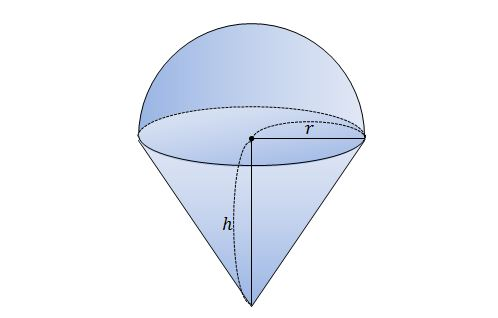If the above diagram consists of a hemi-sphere and a circular cone with $r=9\text{ cm}$ and $h=9\text{ cm},$ what is its volume?

The above diagram is not drawn to scale.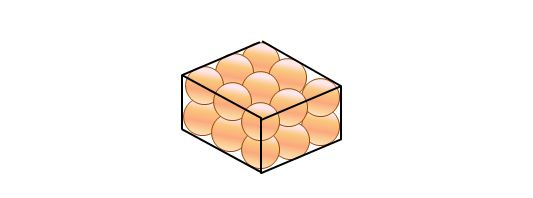$18$ rigid balls of radius $4\text{ cm}$ are closely packed in a rectangular cuboid, as shown above. What is the ratio between the total volume occupied by the $18$ balls and the volume of the rectangular cuboid?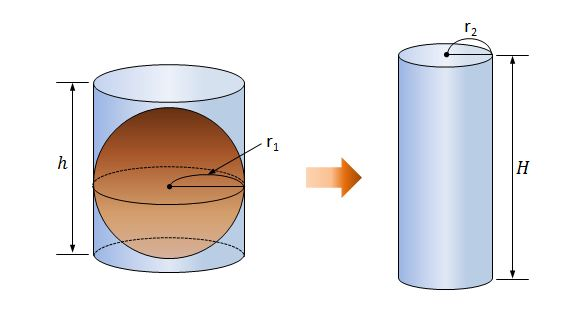The cylinder on the left is filled with water, and the radius and height of the cylinder are $r=3\text{ cm}$ and $h=10\text{ cm},$ respectively. Now, we insert a rigid sphere with radius $3\text{ cm}$ into the cylinder causing the water to spill over. If all the water overflowing from the left cylinder can be contained in the right cylinder with radius $3\text{ cm},$ what is the minimum value of the height $H$ of the right cylinder in $\text{cm} ?$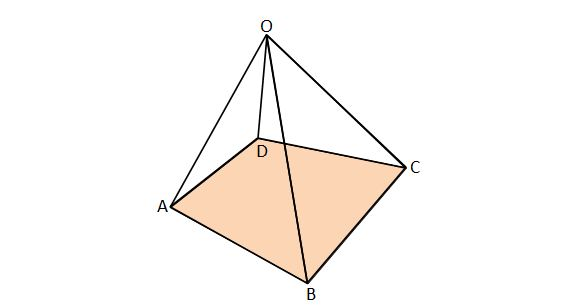The above diagram is a right pyramid with the following lengths: \begin{aligned} {\overline{AB}} &= {\overline{BC}} = {\overline{CD}} = {\overline{AD}} =4, \\ {\overline{OA}} &= {\overline{OB}} = {\overline{OC}} = {\overline{OD}} =2\sqrt{6}. \end{aligned} What is the volume of this pyramid?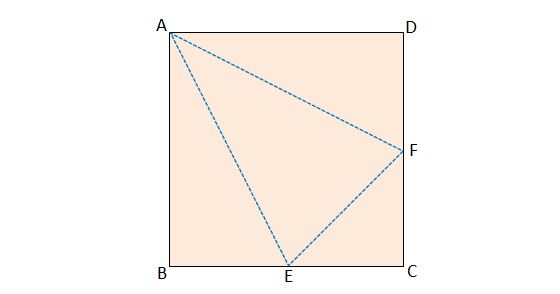Quadrilateral $ABCD$ is a square with side length $18\text{ cm},$ and $E$ and $F$ are the midpoints of $\overline{BC}$ and $\overline{CD},$ respectively. If we fold up the three corners $B,$ $C$ and $D$ to make a polyhedron, then what is the volume of the polyhedron in $\text{cm}^3 ?$

×